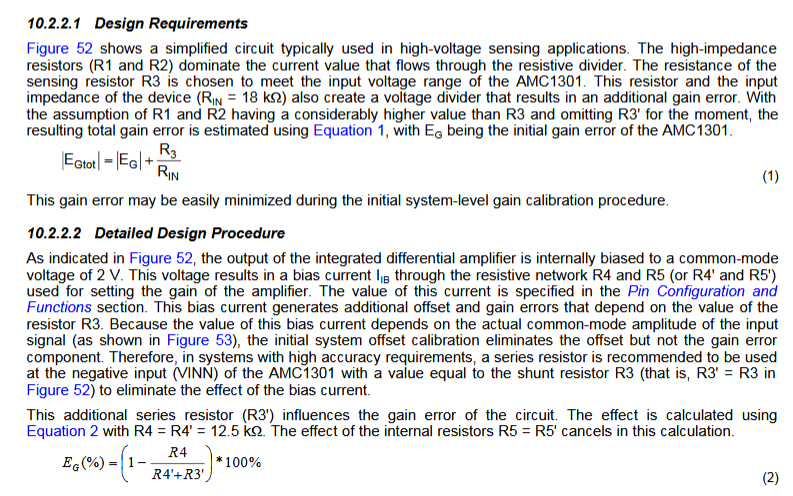If you have a related question, please click the "Ask a related question" button in the top right corner. The newly created question will be automatically linked to this question.

• TI Thinks Resolved

# AMC1301: high precision isolated input

Part Number: AMC1301

Hello,

i want to design a +/-10V input with +/-8V linear inputrange of the AMC1301. Themaximung current of the source is 5mA.

The tolerance of the input must be very low and the bandwidth shoud be a around 50-100kHz.

In the datasheet of the AMC1301 is the following text about gain errors:1. Is it possible to compensate the Gain error according formula (1) by calculating the parallel resistence of the "shuntresistor" and the input resistance of the AMC1301?

2. Is it also possible to compensate the Gain error according formula (2) by using a higher value of R3?

• Hi Jan,

1. That's part of it, doing a 2-point calibration is what would need to be performed.

2. Using a higher value of R3 will actually make the gain error worse since additional current would flow through the input of the device. The goal is to get as much of the current flowing through the shunt resistor as possible.

For this reason, we recommend using high input impedance devices for voltage measurements. To reduce the error in voltage measurement circuits that use a low input impedance device, there are different approaches you can take.

1. Add R3' - this compensates for the bias current that flows through the shunt resistors and creates an offset voltage. While this reduces the offset error significantly, it makes the overall gain error slightly worse. However the overall error is reduced when R3' is used.

2. Perform calibrations. An offset cal, 2-point gain calibration, and/or a 2-point gain calibration over temperature can all be used to reduce the error.

Use the excel calculator shown here to quantify this: https://e2e.ti.com/support/amplifiers/f/14/p/815789/3019110#3019110

I would recommend taking a look at the ISO224 which has a single-ended +/-12V input range, or the AMC1311 which has a 0-2V input range. Both of these devices have high input impedance and are recommended for voltage measurements.

Best regards,

Alex Smith
Applications Engineer | Precision Delta-Sigma Converters